# Gao's theorem for nonabelian groups

 Importance: Medium ✭✭
 Author(s): DeVos, Matt
 Subject: Number Theory » Combinatorial Number Theory
 Keywords: subsequence sum zero sum
 Recomm. for undergrads: no
 Posted by: mdevos on: May 23rd, 2007

For every finite multiplicative group, let(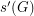) denote the smallest integerso that every sequence ofelements ofhas a subsequence of length(length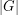) which has product equal to 1 in some order.

Conjecturefor every finite group.

A beautiful theorem of Gao (previously conjectured by Caro) shows that the above property holds for all abelian groups. Rather surprisingly, almost all of the proof for the abelian case seems to work as well for the general case - only one rather innocent looking bit does not carry through. Next we explore this curiosity in detail, beginning with an easy observation.

Observationfor every (finite) group.

To see this, choose a sequence of length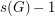of elements which has no nontrivial subsequence with product equal to 1 in any order. Now, appendcopies of 1 to this sequence. The new sequence has lengthand has no subsequence of lengthwith product 1 in any order.

So, the hard part of Gao's theorem is to prove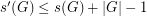, and we now have multiple proofs of this fact. One of the nicest arguments uses a theorem of Kempermann-Scherck, and can be split into the following two parts.

Lemma   Let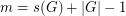and letbe a sequence in an arbitrary finite multiplicativewith the added property that 1 is the most frequently occurring in. Then there is a subsequence ofof lengthwhich has product equal to 1 in some order.
Observation   Ifis a sequence of elements in the finite abelian groupand, then replacing each element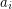ofby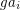has no effect on the products of lengthsubsequences of.

The lemma and observation now combine easily to showin abelian groups, since we may take any sequenceof length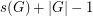and modify it by mutiplying each element by a fixed constant so that 1 is the most common element of. The lemma shows that there is now a subsequence with product 1, and the observation shows that the corresponding subsequence has product 1 in the original. So, surprisingly, the Lemma - which includes all of the real difficutly - works just fine for general groups. The only place we required the assumptionis abelian is for the observation.